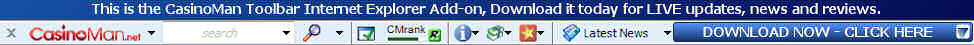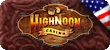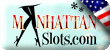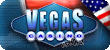06 September 2011, Good Morning

# The Gambling Boundary Problem

Problem: Start with \$c. Place a bet for which you win with probability p <= .50 and lose with probability q = 1 - p. If you win, then you gain \$e; but if you lose the bet, then you pay \$d. Your goal is to reach a top boundary of \$n (or above) before dropping to \$0 (or below). After a fixed number of bets, what are the probabilities of being at each possible dollar amount? In particular, what are the probabilities of reaching your goal and of having gone bust? Lastly, what is the player's average fortune after these fixed number of bets?

Example 1: Suppose we begin with \$6, win with probability p = .38, and receive a payoff of 3 : 2. That is, a loss will cost \$2 and a win will gain \$3. We wish to reach a goal of \$10. After 5 bets, what are the probabilities of having reached \$10 or more and of having gone bust? What is the player's average fortune after these 5 bets?

One can actually reach a fortune of \$12 (if winning while at \$9) and can actually drop to \$-1 (if losing while at \$1). The possible dollar amounts from -1 to 12 create a 14x14 matrix of transition probabilities, denoted by B = [ bij ]. The values bij are conditional probabilities of having \$j after the (k+1)st bet given that one had \$i after the kth bet.

For example, if we had \$8 at some point, then after the next bet we have either \$6 or \$11. Thus, b8, 6 = q and b8,11 = p. Since we quit when reaching \$10 or going bust, b10,10 = b11,11 = b12,12= 1, and b0,0 = b-1,-1 = 1. Here is the complete matrix B for our example:

 -1 0 1 2 3 4 5 6 7 8 9 10 11 12 -1 1 0 0 0 0 0 0 0 0 0 0 0 0 0 0 0 1 0 0 0 0 0 0 0 0 0 0 0 0 1 q 0 0 0 0 p 0 0 0 0 0 0 0 0 2 0 q 0 0 0 0 p 0 0 0 0 0 0 0 3 0 0 q 0 0 0 0 p 0 0 0 0 0 0 4 0 0 0 q 0 0 0 0 p 0 0 0 0 0 5 0 0 0 0 q 0 0 0 0 p 0 0 0 0 6 0 0 0 0 0 q 0 0 0 0 p 0 0 0 7 0 0 0 0 0 0 q 0 0 0 0 p 0 0 8 0 0 0 0 0 0 0 q 0 0 0 0 p 0 9 0 0 0 0 0 0 0 0 q 0 0 0 0 p 10 0 0 0 0 0 0 0 0 0 0 0 1 0 0 11 0 0 0 0 0 0 0 0 0 0 0 0 1 0 12 0 0 0 0 0 0 0 0 0 0 0 0 0 1

The first row and first column represent the possible dollar amounts.

Next, we define A to be the 1x14 initial state matrix which defines the initial dollar amount that occurs with probability 1.

 -1 0 1 2 3 4 5 6 7 8 9 10 11 12 A 0 0 0 0 0 0 0 1 0 0 0 0 0 0

To find the probabilities of having each possible dollar amount after k bets, we multiply A B^k . The probability of having gone ahead after k bets is the sum of the entries in the 10, 11, and 12 dollar amount positions.

To complete the example with p = .38, q = .62, c = 6, n = 10, e = 3, and d = 2, let us determine the probabilities after k = 5 bets. The matrix A B^5 is

 -1 0 1 2 3 4 5 6 7 8 9 10 11 12 ( 0 0.2383 0.1684 0 0 0 0 0.2065 0 0 0 0.1791 0.0632 0.1444 )

Thus, there is a 38.67% chance of reaching the goal of \$10 or more within 5 bets, and a 23.83% chance of going bust.

We now let M be the 1x14 matrix of possible dollar amounts.

 M ( -1 0 1 2 3 4 5 6 7 8 9 10 11 12 )

The average fortune after 5 bets is given by the product A B^5 Transpose[M]. In the above example, this product is \$5.63. Thus after 5 bets, the player will have on average less than the initial amount of \$6.

## Online Gambling Info

• ### Online Casino GamblingName: Email:

### Casino of the Month## You might also like these other casinos:

 High Noon Casino200% First deposit bonus up to \$ 2000 Manhattan Slots100% First deposit bonus up to \$747 Vegas Casino Online100% First deposit bonus up to \$500